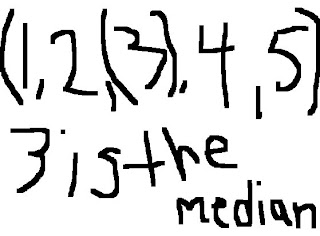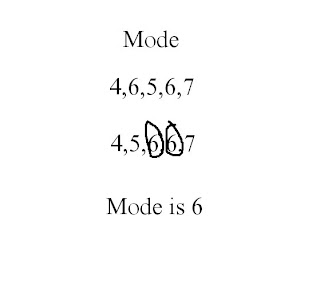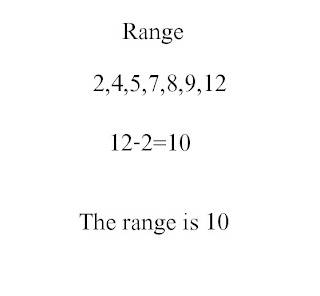### Clarence's Measure of Central Tendency

Thursday, October 2, 2008
Mean : The sum of a value of numbers, divided by the total number of numbers in the list .Median : The smallest number such that at least half the numbers in the list are no greater than it. If the list has an odd number of entries, the median is the middle entry in the list after sorting the list into ascending order. Example : (1,2,(3),4,5) 3 is the median .Mode : The mode is the most common (frequent) value. A list can have more than one mode. A data set has multiple modes when two or more values appear with the same frequency.Range : The positive difference between the largest and smallest values in a set or data .Here is a video that explains MMM